# Trailing 12-Month Usage - How Much? or How Fast?

Follow

Our clients often use Scope-5 trend charts to produce numbers for trailing 12-month resource usage. When using these numbers, it's important to understand that there are different methods for calculating 12-month usage numbers. The two different methods discussed here will mostly yield the same results. However, they will differ very slightly (by ~1/365) around leap-years.

One method (call it the absolute usage method) quantifies the prior 12-month usage by simply adding up the usage numbers from each of the prior 12-months. This method does yield the total usage over the last 12-months but it can be problematic when it’s used to compare performance across different time periods.

To see why, let's imagine that we use the absolute usage method to calculate our 2016 usage (2016 is a leap year) and our 2017 usage. And - let's imagine that in both cases, we come up with 100 MWh. It would be tempting to conclude that we're holding steady in our efficiency - that the identical results indicate that we're using electricity at the same rate in 2017 as we are in 2016. In fact, this is not the case - the numbers tell us that we're actually using electricity very slightly less efficiently in 2017, because the daily rate at which we're using electricity in 2017 is 1/365th higher (we used the same amount of energy in 2017 in 365 days as we did in 2016 in 366 days).

Scope 5 is concerned first and foremost with helping our clients understand the rate at which they use resources rather than the absolute amount they use in any time period. For this reason, Scope-5 uses a slightly different method to produce numbers for trailing 12-month resource usage (call it the usage rate method).

Scope 5 trend charts can be configured to calculate an annual rate for the trailing 12 months, as shown below: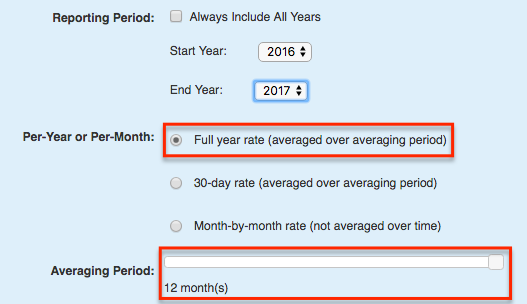These configuration parameters tell Scope 5 to calculate at each point in time, the annual (full-year) usage rate over the last 12-months. To do so, Scope 5 applies the usage rate method. This method will produce the same results as the absolute usage method if the trailing 12 months don’t span a February 29th. If they do span a February 29th, the results will differ slightly. To see how, let's apply the two different calculation methods to the 100MWh example.

First, for 2017 - using the absolute usage method, the annual usage for the trailing 12 months is generated simply by summing the usage over the prior 12-months: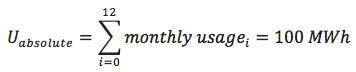Scope 5 starts with the same prior 12-month absolute usage number to calculate average daily usage for 2017: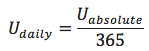Next, it standardizes to a 365-day year by multiplying the daily rate by 365: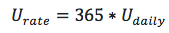For 2017, the two methods yield the same result: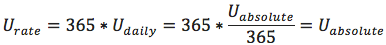Now let’s see how this works for 2016. This time, daily usage is calculated by dividing the prior 12-month usage by 366 (not 365):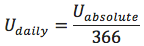That daily rate is then standardized to a 365 day year by multiplying by 365: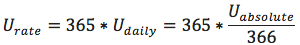or: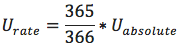The number produced by the usage rate method is then slightly lower for 2016 than the total annual usage produced by the absolute usage method. Using the 100 MWh number: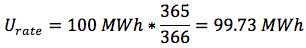In summary, though the difference between the two results is minimal, it’s important to understand that both methods yield valid results. The usage rate method is better suited for comparisons across time periods. The absolute usage method is better suited for traditional accounting purposes.